# JEE Advanced 2020 Physics Paper 1

JEE Advanced 2020 Question Paper for Physics (Paper 1) had 18 questions and 66 marks weightage was given. Students will find all the questions asked in the exam and they have been solved by our experts. Meaning, students have the benefit of learning the answers right here and this will further enable them to practice and comprehend every question easily even by just taking a glance. Students can therefore use the question paper to practice and solve questions easily. They will be able to study more effectively and be ready to crack the different types of questions that may be asked in the entrance exam.

### Paper 1 - Physics

Question 1. A football of radius R is kept on a hole of radius r(𝑟<𝑅) made on a plank kept horizontally. One end of the plank is now lifted so that it gets tilted making an angle θ from the horizontal as shown in the figure below. The maximum value of θ so that the football does not start rolling down the plank satisfies (the figure is schematic and not drawn to scale)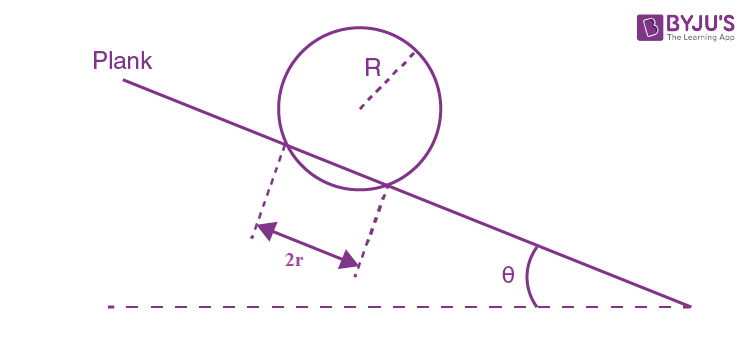1. a) sin θ = r / R
2. b) tan θ = r / R
3. c) sin θ = r / 2R
4. d) cos θ = r / 2R

Solution:

Draw free body diagram, where the normal reaction will act on point of contact. So,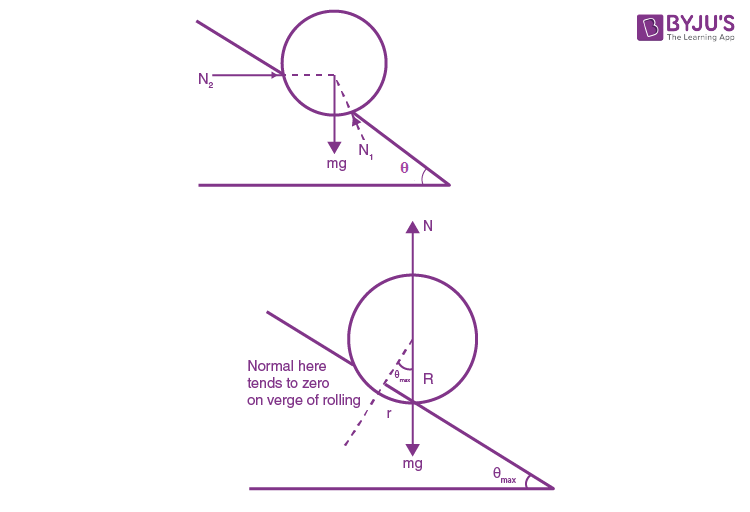In the limiting case, mg will act along a normal reaction. For max θ, the football is about to roll, then N2 = 0 and all the forces (mg and N1) must pass through the contact point. So,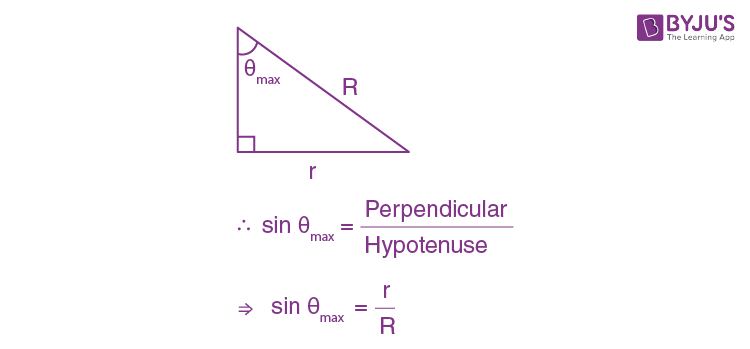Question 2. A light disc made of aluminium (a nonmagnetic material) is kept horizontally and is free to rotate about its axis as shown in the figure. A strong magnet is held vertically at a point above the disc away from its axis. On revolving the magnet about the axis of the disc, the disc will (the figure is schematic and not drawn to scale);1. a) rotate in the direction opposite to the direction of magnet’s motion
2. b) rotate in the same direction as the direction of magnet’s motion
3. c) not rotate and its temperature will remain unchanged
4. d) not rotate but its temperature will slowly rise

Solution:By Lenz's law, the disc also tries to move in the same direction because in the backward part of the disc the flux reduces as magnet moves and as there is a change in magnetic flux, so there is eddy current production which leads to the production of heat. These currents are such that it opposes the relative motion. So, the disc will rotate in the direction of rotation of the magnet.

Question 3. A small roller of diameter 20 cm has an axle of diameter 10 cm (see figure below on the left). It is on a horizontal floor and a meter scale is positioned horizontally on its axle with one edge of the scale on top of the axle (see figure on the right). The scale is now pushed slowly on the axle so that it moves without slipping on the axle, and the roller starts rolling without slipping. After the roller has moved 50 cm, the position of the scale will look like (figures are schematic and not drawn to scale);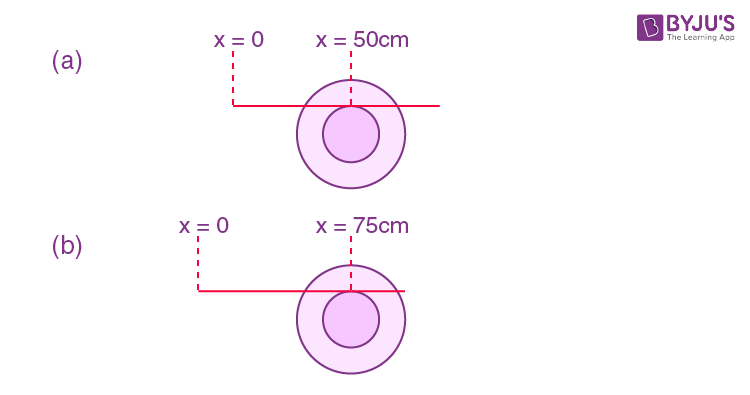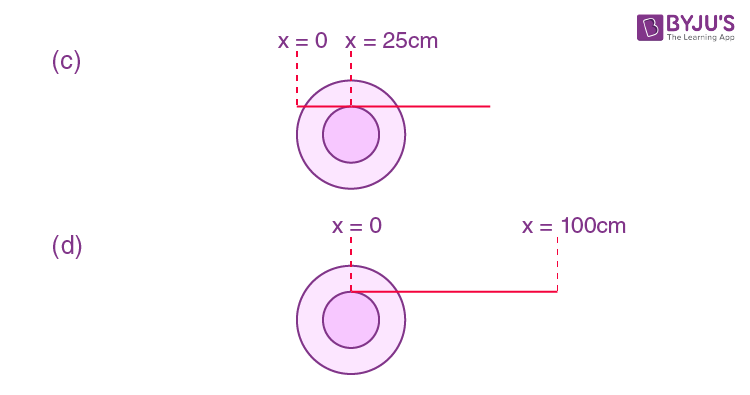Solution:

∵ In this case pure rolling without slipping,

Let radius of roller is R and radius of axle is r.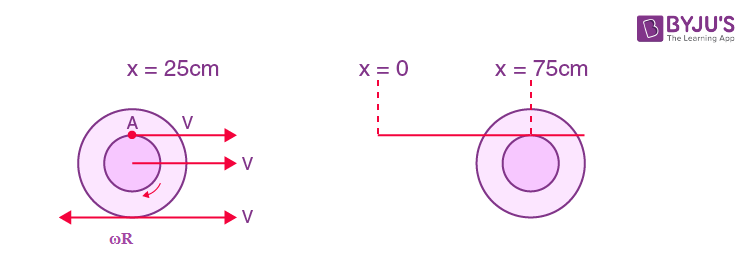∵ As we know, v = ω r

Now from the figure,

V – ωR = 0

V = ωR

v = 10 ω ⇒ ω = v / 10

∵ Radius = Diameter / 2

Velocity of point (A) = v + ωr

Now, put the values of ω and r,

= v + v / 10 X 5

= 3v / 2 = 1.5v

So, distance moved by the point of contact

= 1.5 X 50 cm = 75 cm

Question 4. A circular coil of radius R and N turns has negligible resistance. As shown in the schematic figure, its two ends are connected to two wires and it is hanging by those wires with its plane being vertical. The wires are connected to a capacitor with charge Q through a switch. The coil is in a horizontal uniform magnetic field Bo parallel to the plane of the coil. When the switch is closed, the capacitor gets discharged through the coil in a very short time. By the time the capacitor is discharged fully, magnitude of the angular momentum gained by the coil will be (assume that the discharge time is so short that the coil has hardly rotated during this time)1. a) 𝜋/2 𝑁𝑄𝐵𝑜𝑅2
2. b) 𝜋𝑁𝑄𝐵𝑜𝑅2
3. c) 2𝜋𝑁𝑄𝐵𝑜𝑅2
4. d) 4𝜋𝑁𝑄𝐵𝑜𝑅2

Solution: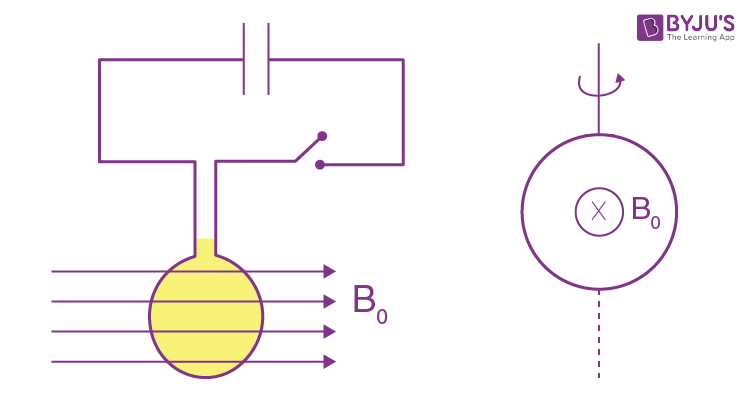After closing switch, within a fraction of seconds, entire charge flows through the coil and produces impulsive torque.

Now, change in Angular momentum ⇒

∫ 𝜏 dt = ∆L ……(1)

$$\vec{\tau} = \vec{M} \times \vec{B}$$

Where,

$$\vec{M}$$
= Magnetic moment

$$\vec{B}$$
= Magnetic field

$$\vec{M}$$
= Ni (πR2)

∵ 𝜏 = MB sin90° ⇒ MB ⇒ (NiπR2) Bo

∵ sin 90° = 1

Put all the values in equation (1),

∫ NiπR2Bodt = ∆L

⇒ NπR2 Bo ∫ idt = ∆L

According to question ∫ idt = Q

NπR2 BoQ = ∆L

πNQBoR2 = ∆L

Question 5. A parallel beam of light strikes a piece of transparent glass having cross section as shown in the figure below. Correct shape of the emergent wavefront will be (figures are schematic and not drawn to scale)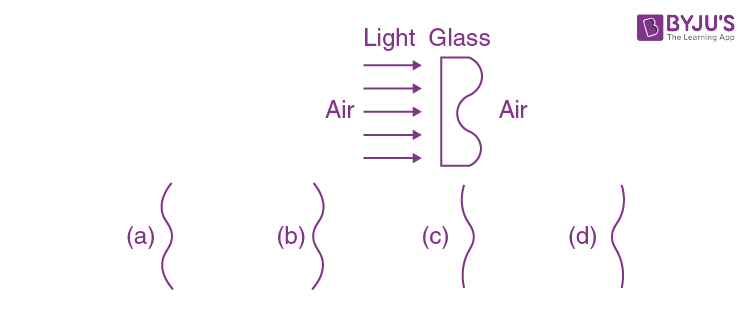Solution: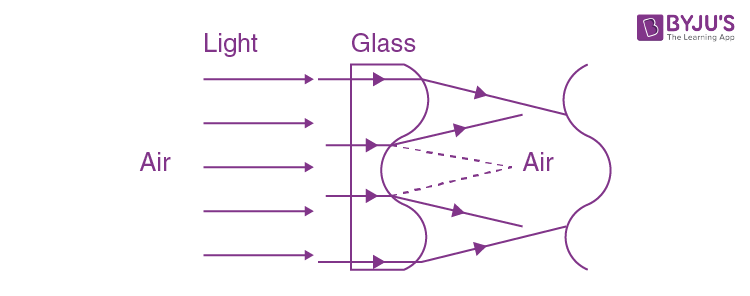Concept: based on Huygens’ principle.

Theory of wavefront → wave moves such that direction of propagation is perpendicular to the wavefront.

And as we can see that light ray will travel more in air than glass medium. And if the glass medium is more than wavefront will slack. So, the shape of the emergent wavefront will beQuestion 6. An open-ended U-tube of uniform cross-sectional area contains water (density 103kg m−3). Initially, the water level stands at 0.29 m from the bottom in each arm. Kerosene oil (a water-immiscible liquid) of density 800 kg m−3 is added to the left arm until its length is 0.1 m, as shown in the schematic figure below. The ratio h2 / h2 of the heights of the liquid in the two arms is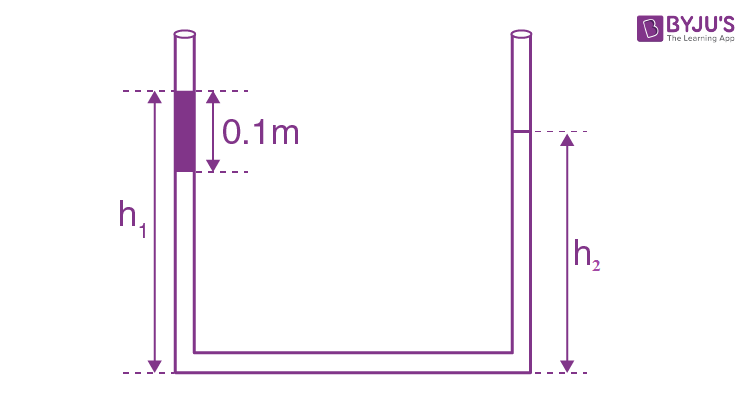1. a) 15 / 14
2. b) 35 / 33
3. c) 7 / 6
4. d) 5 / 4

Solution: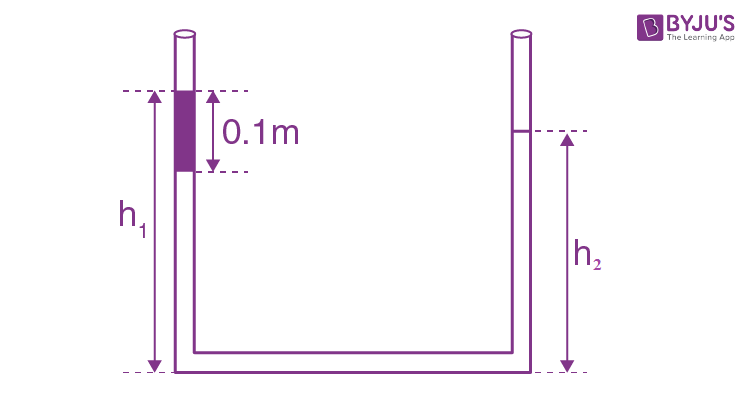∵ Pressure will be same at same horizontal level,

PA = PB (Same horizontal level)

Patm + ρkero g x 0.1 + ρw g(h1 – 0.1) = Patm + ρwgh2

Where, Patm = Atmospheric pressure

ρkero = density of kerosene = 800 kg/m3

ρw = density of water = 1000 kg/m3

g = acceleration due to gravity.

As we know, pressure force due to liquid column = ρgh

⇒ 800x 0.1 + 1000 (h1 - 0.1) = 1000h2

⇒ 0.8 + 10h1 – 1 = 10h2

⇒ 10(h1 – h2) = 0.2 ….....(1)

h1 – h2 = 0.02

Also, initial level of water in both arms = 2×0.29

Therefore,

h1 – 0.1 + h2 = 0.58

h1 + h2 = 0.68 ….....(2)

From (1) & (2)

h1 = 0.35 and h2 = 0.33

So, h1 / h2 = 35 / 33

Question 7. A particle of mass m moves in circular orbits with potential energy V(𝑟) = 𝐹𝑟, where F is a positive constant and r is its distance from the origin. Its energies are calculated using the Bohr model. If the radius of the particle’s orbit is denoted by R and its speed and energy are denoted by v and E, respectively, then for the nth orbit (here h is the Planck’s constant)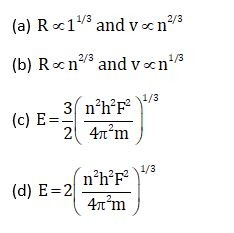Solution:

P.E., V(r) = Fr

Therefore,

Force = -dV/dr= – F

F = mv2 / R …. (1)

MvR = nh / 2 π ….. (2)

From (2) R = nh / 2 πmv

R = kn / n1/3

R α n2/3

E = KE + PE

$$\frac{1}{2}mv^{2} + V(r) = \frac{1}{2}FR+FR$$

$$\frac{3}{2} FR = \frac{3}{2}F \times \frac{nh}{2\pi m(\frac{nFh}{2\pi m^{2}})^{\frac{1}{3}}}$$

$$E = \frac{3}{2}\left ( \frac{n^{2}h^{2}F^{2}}{4\pi ^{2}M} \right )^{\frac{1}{3}}$$

Option b and c.

Question 8. The filament of a light bulb has a surface area 64 mm2. The filament can be considered as a black body at temperature 2500 K emitting radiation like a point source when viewed from far. At night the light bulb is observed from a distance of 100 m. Assume the pupil of the eyes of the observer to be circular with radius 3 mm. Then (Take Stefan-Boltzmann constant = 5.67 × 10−8 Wm−2K−4,

Wien’s displacement constant = 2.90 × 10−3m-K,

Planck’s constant = 6.63 × 10−34Js,

speed of light in vacuum = 3.00 ×108 ms−1)

1. a) power radiated by the filament is in the range 642 W to 645 W
2. b) radiated power entering into one eye of the observer is in the range 3.15 × 10−8 W to 3.25 × 10−8 W
3. c) the wavelength corresponding to the maximum intensity of light is 1160 nm
4. d) taking the average wavelength of emitted radiation to be 1740 nm, the total number of photons entering per second into one eye of the observer is in the range 2.75 × 1011 to 2.85 × 1011

Solution:

Surface area of filament (A) = 64 mm2

Temperature (T) = 2500K

P = e σAT4

= 1 X 5.67 X 10-8 X 64 X 10-6 X (2500)4 = 141.75 W

Radiated power entering one's eye =

$$\frac{P}{4\pi R^{2}}\times \pi r^{2}$$

$$\frac{141.75\times (3\times 10^{-3})^{2}}{4(100)^{2}} = 318.9 \times 10^{-10}$$

= 3.19×10-8 W → option (b)

For wavelength corresponding to maximum intensity,

λm T = B

$$\lambda _{m} = \frac{2.93\times 10^{6}}{2500}$$
= 1160nm → option (c)

For no of photons, per sec,

$$N\frac{hc}{\lambda } = P$$

$$N\frac{P\lambda }{hc} = \frac{3.19 \times 10^{-8} \times 1740 \times 10^{-9}}{6.67 \times 10^{-34} \times 3 \times 10^{8} } = 2.77\times 10^{11} Option (d).$$

Ans. b, c, d

Question 9. Sometimes it is convenient to construct a system of units so that all quantities can be expressed in terms of only one physical quantity. In one such system, dimensions of different quantities are given in terms of a quantity X as follows: [position] = [𝑋𝛼]; [speed] = [𝑋𝛽]; [acceleration] =[𝑋𝑝]; [linear momentum] = [𝑋𝑞]; [force] = [𝑋𝑟]. Then

1. a) α + 𝑝 = 2β
2. b) 𝑝 + 𝑞 − 𝑟 = β
3. c) − 𝑞 + 𝑟 = α
4. d) 𝑝 + 𝑞 + 𝑟 = β

Solution:

Position = [x𝛼]

⇒ [L] = [𝑋𝛼] ..... (1)

speed = x𝛽

LT-1 = 𝑋𝛽

𝑋𝛼 T-1 = X𝛽 (from (1)

T-1 = 𝑋𝛼-𝛽….. (2)

Acceleration = XP

LT-1 × T-1 = Xp

𝑋𝛽.𝑋𝛼-𝛽 = xp

𝑋2𝛼-𝛽 = xp

⇒ 2β – α = p

α + p = 2β

Option (a)

[Linear momentum] = xq

M LT-1 = xq

M.X 𝛽 = xq

M = xq-𝛽 …... (3)

[force] = xr

M LT-2 = xr

M.Xp = xr

M = xr-p …..(4)

From (3) and (4), q - β = r - p

p + q - r = β option (B)

From (a) p + q -r = α / 2 + p / 2

P / 2 + q - r = α / 2

Ans: a, b

Question 10. A uniform electric field,

$$\vec{E} = -400\sqrt{3\hat{y}}NC^{-1}$$
is applied in a region. A charged particle of mass m carrying positive charge q is projected in this region with an initial speed of
$$2\sqrt{10} \times 10^{6} ms^{-1}$$
. This particle is aimed to hit a target T, which is 5 m away from its entry point into the field as shown schematically in the figure. Take q / m = 1010CKg-1. Then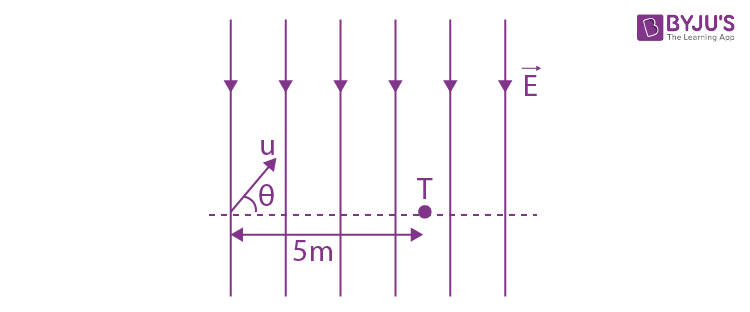1. a) the particle will hit T if projected at an angle 45o from the horizontal
2. b) the particle will hit T if projected either at an angle 30o or 60o from the horizontal
3. c) time taken by the particle to hit T could be
$$\sqrt{\frac{5}{6}}\mu s$$
as well as
$$\sqrt{\frac{5}{2}}\mu s$$
4. d) time taken by the particle to hit T is
$$\sqrt{\frac{5}{3}}\mu s$$

Solution: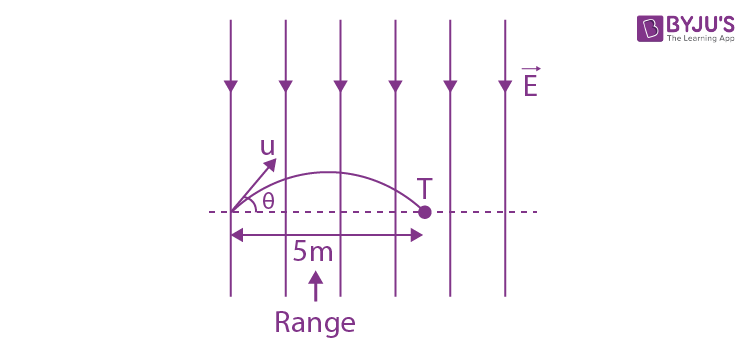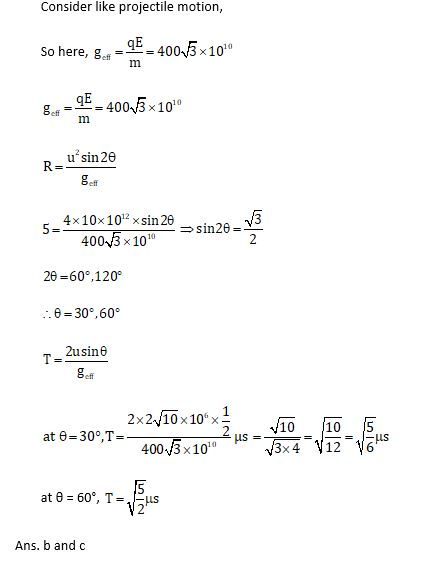Question 11. Shown in the figure is a semicircular metallic strip that has thickness t and resistivity ρ. Its inner radius is R1 and outer radius is R2. If a voltage V0 is applied between its two ends, a current I flows in it. In addition, it is observed that a transverse voltage ∆𝑉 develops between its inner and outer surfaces due to purely kinetic effects of moving electrons (ignore any role of the magnetic field due to the current). Then (figure is schematic and not drawn to scale)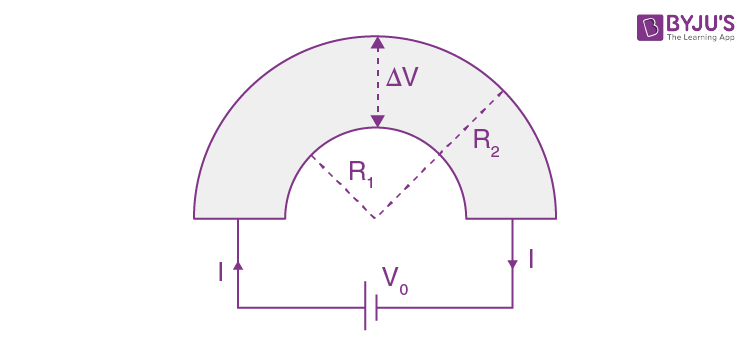1. a)
$$I = \frac{v_{0}t}{\pi \rho }1n\left ( \frac{R_{1}}{R_{2}} \right )$$
2. b) the outer surface is at a higher voltage than the inner surface
3. c) the outer surface is at a lower voltage than the inner surface
4. d) ∆𝑉α𝐼2

Solution:

To calculate resistance, ℓ = πr, A = tdr

dr = ρ πr / tdr

But all elemental strips are in parallel so,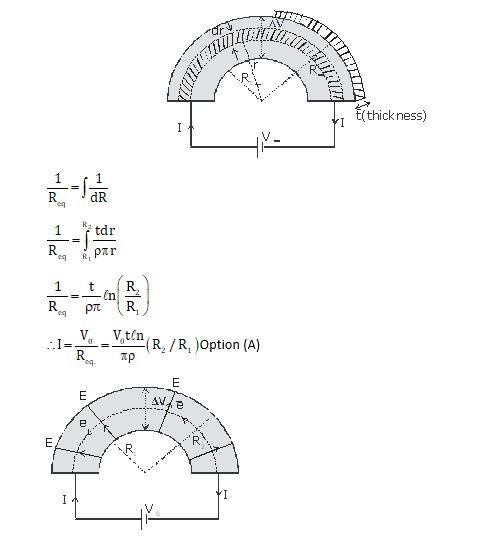Electrons move in a circular path so they exp. centripetal force due to the electric field set up because of p.d., so 'E' field should be from inner to outer, therefore the outer surface is at a lower voltage than inner.

To check p.d. across the surface, let us consider elemental resistance

dr = ρ πr / tdr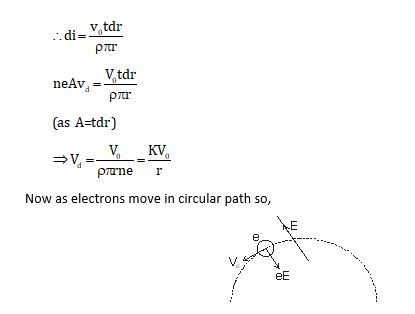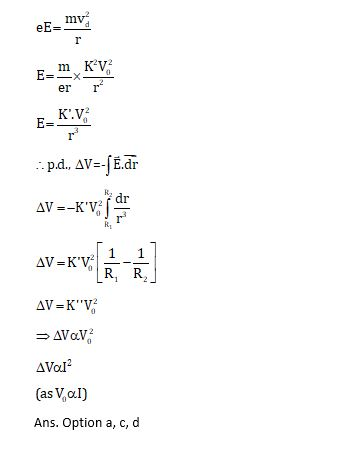Question 12. As shown schematically in the figure, two vessels contain water solutions (at temperature T) of potassium permanganate (KMnO4) of different concentrations 𝑛1 and 𝑛2(𝑛1 >𝑛2) molecules per unit volume with ∆𝑛 = (𝑛1 −𝑛2) ≪ 𝑛1. When they are connected by a tube of small length l and cross-sectional area S, KMnO4 starts to diffuse from the left to the right vessel through the tube. Consider the collection of molecules to behave as dilute ideal gases and the difference in their partial pressure in the two vessels causing the diffusion. The speed v of the molecules is limited by the viscous force −𝛽𝑣 on each molecule, where β is a constant. Neglecting all terms of the order (∆𝑛)2, which of the following is/are correct? (𝑘𝐵 is the Boltzmann constant)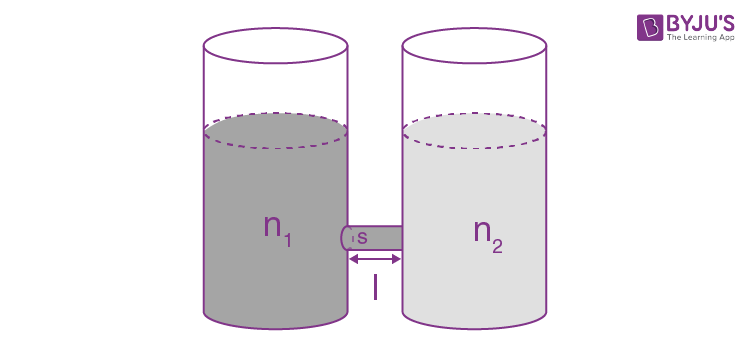1. a) the force causing the molecules to move across the tube is ∆𝑛𝑘𝐵𝑇𝑆
2. b) force balance implies 𝑛1𝛽𝑣𝑙 = ∆𝑛𝑘𝐵𝑇
3. c) total number of molecules going across the tube per sec is
$$\left ( \frac{\Delta n}{I} \right )\left ( \frac{k_{\beta }T}{\beta} \right )S$$
4. d) rate of molecules getting transferred through the tube does not change with time

Solution: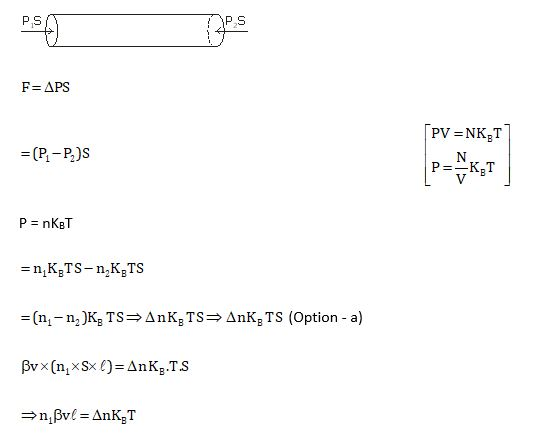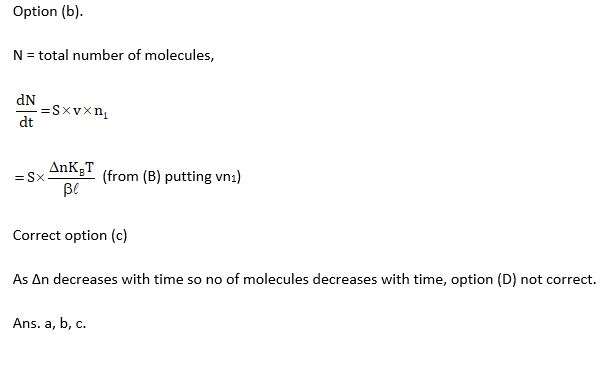Question 13. Put a uniform meter scale horizontally on your extended index fingers with the left one at 0.00 cm and the right one at 90.00 cm. When you attempt to move both the fingers slowly towards the centre, initially only the left finger slips with respect to the scale and the right finger does not. After some distance, the left finger stops and the right one start slipping. Then the right finger stops at a distance 𝑥𝑅 from the centre (50.00 cm) of the scale and the left one starts slipping again. This happens because of the difference in the frictional forces on the two fingers. If the coefficients of static and dynamic friction between the fingers and the scale are 0.40 and 0.32, respectively, the value of 𝑥𝑅 (in cm) is ______.

Solution: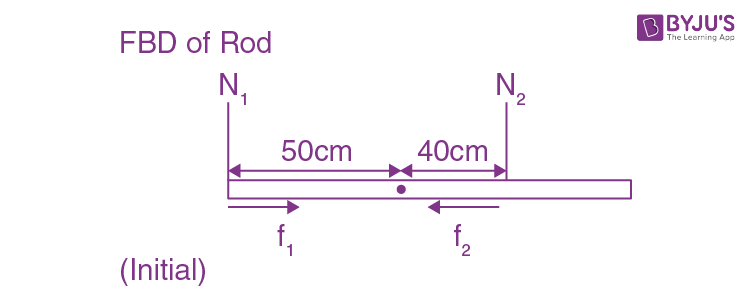As the rod is in equilibrium

∴ 50N1 = 40 N2

∴ N2 > N1

∴ by balancing torque, we conclude N2>N1, so f2>f1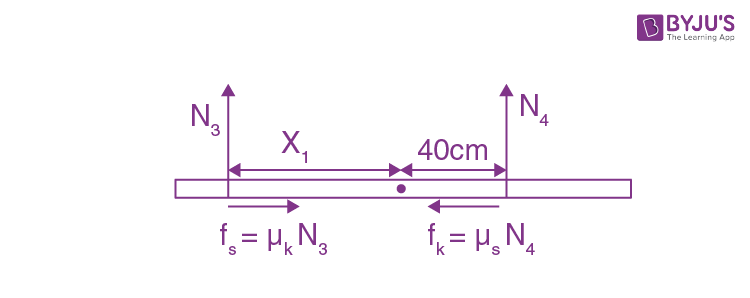As rod does not move, only left finger slips,

So Fnet = 0 μKN3 = μSN4

0.32N3 = 0.40 N4 ..... (1)

𝜏netabout centre = 0, x1N3 = 40N4 ..... (2)

Dividing eq. (1) by (2)

0.32 / x1 = 0.40 / 40 ⇒ x1 = 32cm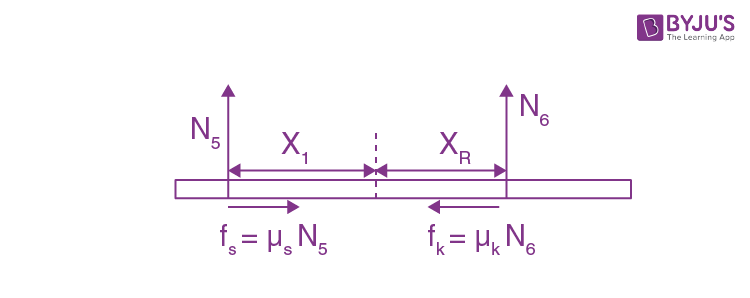= Fnet = 0, μ sN5 = μkN6

0.40N5 = 0.32N6 ..... (3)

𝜏net = 0, N5x1 = N6 xR ..... (4)

Div eq.(3) by eq.(4),

0.40 / x1 = 0.32 / xR

0.40 / 32 cm = 0.32 / xR

⇒ XR = 32 X 32 / 40 = 128 / 5 = 25.60 cm

Ans. 25.60

Question 14. When water is filled carefully in a glass, one can fill it to a height h above the rim of the glass due to the surface tension of water. To calculate h just before water starts flowing, model the shape of the water above the rim as a disc of thickness h having semicircular edges, as shown schematically in the figure. When the pressure of water at the bottom of this disc exceeds what can be withstood due to the surface tension, the water surface breaks near the rim and water starts flowing from there. If the density of water, its surface tension and the acceleration due to gravity are 103kg m−3, 0.07 Nm−1 and 10 ms−2, respectively, the value of h (in mm) is _________.

Solution:Let radius of disc = r

[A = projected area = h(2r)]

(After balancing effect of Patm on both sides)S × 2πr = Pavg. × h(2πr)

Pavg. = 0 + ρgh / 2 = ρgh / 2

S = ( ρgh / 2) X h

2S / ρg = h2

$$\Rightarrow h = \sqrt{\frac{2S}{\rho g}} = \sqrt{\frac{2\times 0.07}{10^{3}\times 10}} = \sqrt{14}\times 10^{-3} = 3.74mm$$

Ans = 3.74

Question 15. One end of a spring of negligible unstretched length and spring constant k is fixed at the origin (0, 0). A point particle of mass m carrying a positive charge q is attached at its other end. The entire system is kept on a smooth horizontal surface. When a point dipole 𝑝 pointing towards the charge q is fixed at the origin, the spring gets stretched to a length l and attains a new equilibrium position (see figure below). If the point mass is now displaced slightly by ∆𝑙 ≪ 𝑙from its equilibrium position and released, it is found to oscillate at frequency

$$\frac{1}{\delta }\sqrt{\frac{k}{m}}$$
. The value of δ is ______.

Solution:As we know, for spring block system,

$$f = \frac{1}{2\pi}\sqrt{\frac{k}{m}}$$
,

So we can also observe 'k' as

$$\frac{d^{2}U}{dx^{2}}$$
from eq.

$$U = \frac{1}{2}kx^{2}$$

$$\frac{du}{dx} = Kx$$

$$\frac{d^{2}U}{dx^{2}} = K$$

So, we can say,

$$f = \frac{1}{2\pi}\sqrt{\frac{\frac{d^{2}U}{dx^{2}}}{m}}$$

The above concept let us find Potential Energy, (U) of system from equilibrium.

As given, negligible unstretched length, so spring length is elongated length only.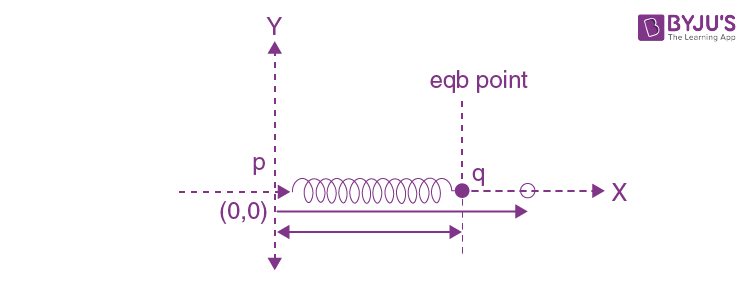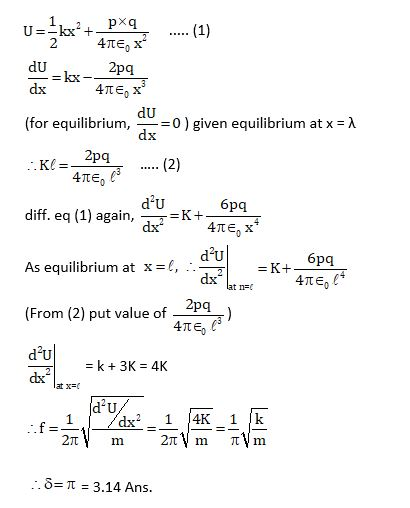Question 16. Consider one mole of helium gas enclosed in a container at initial pressure 𝑃1 and volume 𝑉1. It expands isothermally to volume 4𝑉1. After this, the gas expands adiabatically and its volume becomes 32𝑉1. The work done by the gas during isothermal and adiabatic expansion processes are 𝑊𝑖𝑠𝑜 and 𝑊𝑎𝑑𝑖𝑎, respectively. If the ratio 𝑊𝑖𝑠𝑜 / 𝑊𝑎𝑑𝑖𝑎 = fln2, then f is ________.

Solution:

Let the initial temperature be T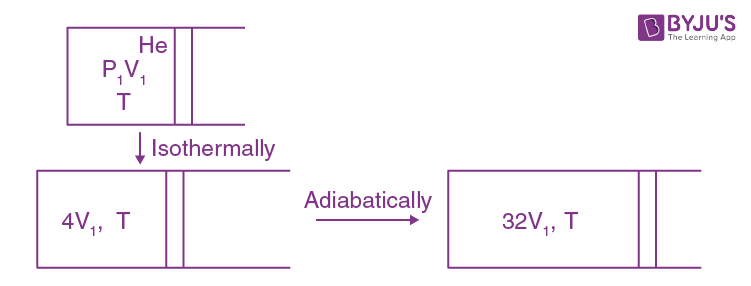TV γ -1 = Constant, γ = 5 / 3

$$T_{2} = T_{1}\left ( \frac{4V_{1}}{32V_{1}} \right )^{\frac{5}{3}-1}$$

$$= T_{1}\left ( \frac{1}{8} \right )^{\frac{2}{3}}$$

$$= \frac{T_{1}}{4}$$

$$\frac{nR(T_{1}-T_{2})}{\gamma -1}$$

=

$$\frac{nR(T_{1}-\frac{T_{1}}{4})}{\frac{5}{3} -1}$$

=

$$\frac{3}{2}nR \times \frac{3T_{1}}{4} = \frac{9}{8}nRT_{1}$$

Now work done in isothermal;

Wiso =

$$nRT_{1}In\left ( \frac{V_{2}}{V_{1}} \right )$$

=

$$nRT_{1}In\left ( \frac{4V_{1}}{V_{1}} \right )$$

= 2nRT_{1} ln2

$$\frac{W_{iso}}{W_{adb}} = \frac{2nRT_{1} ln2}{\frac{9}{8}nRT_{1}}$$

=

$$\frac{16}{9}ln2$$

∴ f = 16 / 9 = 1.77

Question 17. A stationary tuning fork is in resonance with an air column in a pipe. If the tuning fork is moved with a speed of 2 ms−1 in front of the open end of the pipe and parallel to it, the length of the pipe should be changed for the resonance to occur with the moving tuning fork. If the speed of sound in air is 320 ms−1, the smallest value of the percentage change required in the length of the pipe is ____________.

Solution:

For open pipe resonance, f = nv / 4L

Let us consider for n = 1,

Initial length of pipe = L1 for f1

$$\left ( f_{1} = \frac{V}{4L_{1}} \right ) ......(1)$$

Now due to Doppler’s effect,

$$f_{2} = \left ( \frac{320}{320-2} \right )f_{1}$$

$$f_{2} = \left ( \frac{320}{320-2} \right )f_{1}$$

$$f_{2} = \frac{v}{4L_{2}} \, \; .....(3)$$

$$\frac{320}{318} \times \frac{V}{4L_{1}} = \frac{V}{4L_{2}}$$

$$\frac{L_{1}}{L_{2}} = \frac{318}{320}$$

% change in length,

$$\frac{\Delta L}{L}\times 100% = \left ( \frac{L_{1}-L_{2}}{L_{1}} \right )\times 100%$$

=

$$\left ( 1-\frac{L_{2}}{L_{1}} \right )\times 100%$$

=

$$\left ( 1-\frac{318}{320} \right )\times 100%$$

=

$$\frac{200}{320}% = \frac{5}{8}% = 0.0625%$$

= 0.62%

Ans. 0.62

Question 18. A circular disc of radius R carries surface charge density

$$\sigma (r) = \sigma _{o}\left ( 1-\frac{r}{R} \right )$$
, where 𝜎0 is a constant and r is the distance from the center of the disc. Electric flux through a large spherical surface that encloses the charged disc completely is 𝜙0. Electric flux through another spherical surface of radius R/4 and concentric with the disc is 𝜙. Then the ratio
$$\frac{\phi_{o}}{\phi }$$
is _________.

Solution:Charge in elemental ring = ∫ rr=0 σ2πr dr

φ0 = qenc/ ε0 (charges enclosed completely, so r = R)

and φ = qenc/ ε0 (…upto r = R/4)

q = ∫ r0 σ0 (1-r/R) 2πr dr

= 2π σ0 r0 r dr - (2πσ0/R) ∫ r0 r2 dr

= 2π σ0 [r2/2 - r3/3R)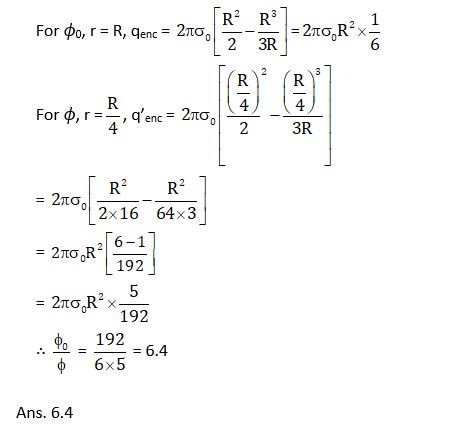### JEE Advanced 2020 Physics Paper 1 Solutions